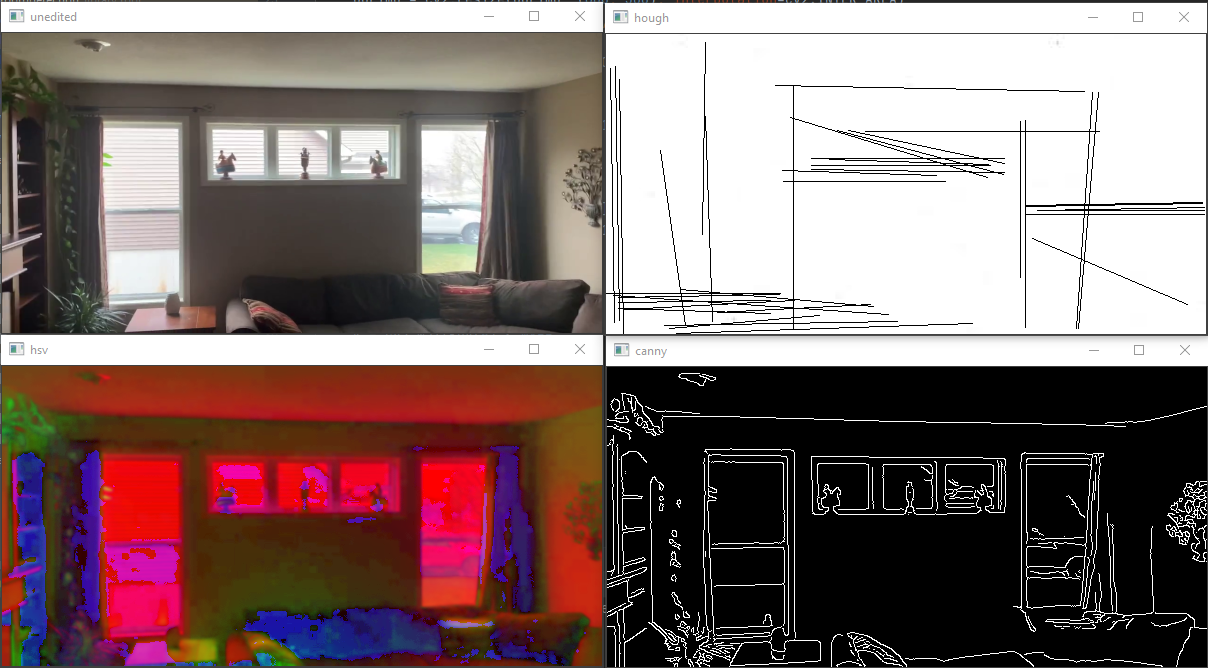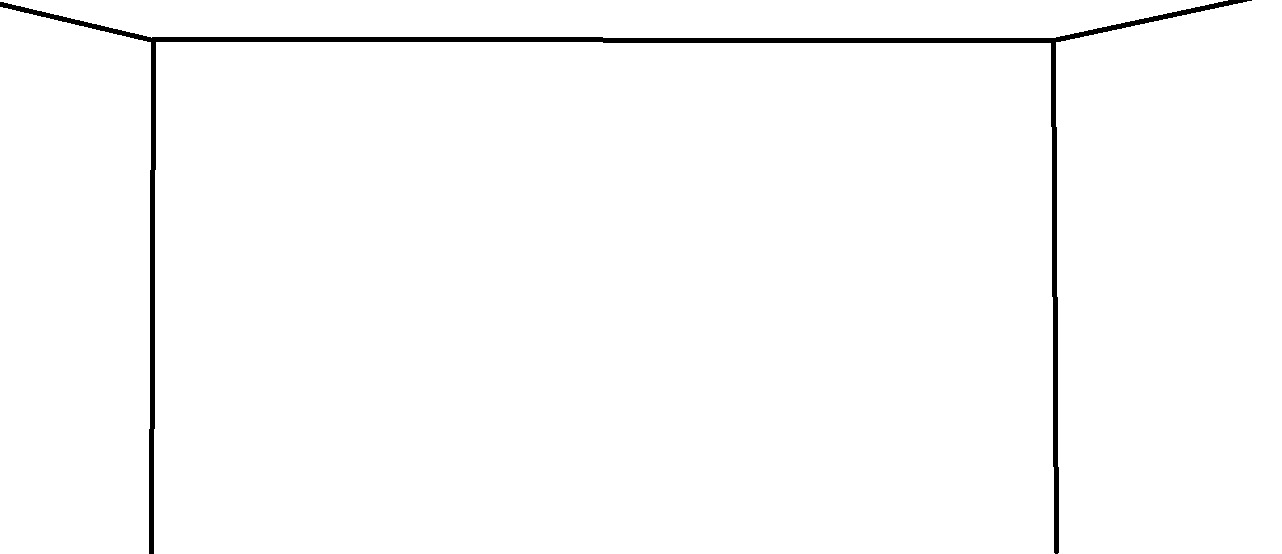#### How can I remove noise with Hough Line Transform with opencv-python

The purpose of this program is to map out the corners and edges of a room, here is where I’m at right now:The image on the top right is my result, and the image on the top left is my input. Currently, I am using canny edge detection with a gaussian filter. But as you can see, there are a lot of random lines coming from other details in the image.

My goal is this:So far, I’ve tried,
Only showing lines that are flat (0 or infinite slope):

``````if lines is not None:
for line in lines:
x1, y1, x2, y2 = line
if math.isclose(x1, x2, tol_abs=25) or math.isclose(y1, y2, tol_abs=10):
cv2.line(bgrimg, (x1, y1), (x2, y2), (0, 255, 0), 1)
``````

But this resulted in me losing some of the important lines.
Next, I tried to only show lines on the edges of the image:

``````if lines is not None:
for line in lines:
x1, y1, x2, y2 = line
if y1 > 100 and y2 > 100:
cv2.line(bgrimg, (x1, y1), (x2, y2), (0, 255, 0), 1)
``````

This somewhat works for the top, but since the sides could be anywhere in the picture, I can’t determine exactly where they will be.

Is there a way to solve this? If not, would it be possible with machine learning?

Source: Python Questions